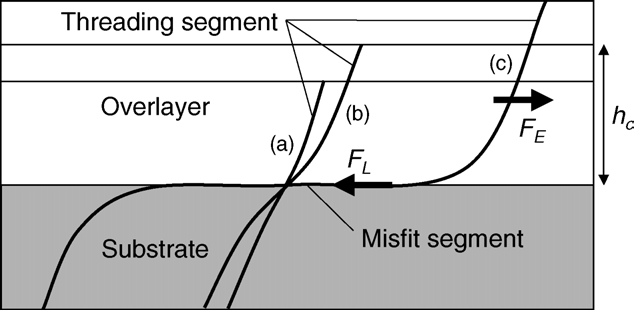# Critical Thickness

In the previous discussion, the notion of a critical thickness has been introduced but has not been discussed profoundly. One of the first quantitative models of dislocations was that of Frank and van der Merwe. Instead of the stress tensor defined above, Frank and van der Merwe defined a string of discrete particles bound by a Hooke's law-type potential and a sinusoidal background lattice potential. They then took the continuum limit but kept the interatomic distance fixed. Effectively this means intrapolating the spatial deviations$\xi(x)$ (perpendicular to the string) of the atom at location x (along the string) by a continuous function. Minimising the potential with respect to$\xi$ and solving for$\xi$ yields the static distribution of perpendicular spatial atom deviations as a function of position on the string. A dislocation is then defined to be large variations in$\xi$ for short intervals in x. With this model suitable for screw dislocations, one can calculate the critical thickness:$h_c=\frac{b}{8 \pi (1+\nu)(|\epsilon|+\frac{b}{d})} (1+\log(\frac{2 h_c}{r}))$

Here, b is the magnitude of the Burger's vector,$\nu$ the Poisson ratio,$\epsilon$ the elastic strain as defined above and r the inner cut-off radius of the misfit dislocation. A force balance consideration between the tension force along the dislocation and the stress due to the missfit by Matthews and Blakeslee yields a result very similar in functional form. See figure: